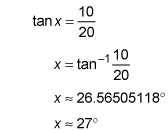##### Geometry: 1,001 Practice Problems For Dummies (+ Free Online Practice)In geometry, if you’re given a right triangle with missing angles or sides, you can use trigonometric ratios—sine, cosine, or tangent—to find them.

To help you decide which of the three trigonometric ratios to use, you can label the sides of the triangle as adjacent or opposite. This labeling is dependent on the given angle in the right triangle. The hypotenuse is always the side opposite the right angle.

The following practice questions ask you to solve problems using the cosine and tan functions.

## Practice questions

1. Triangle CST,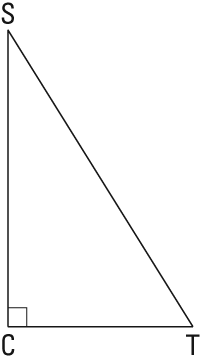is a right triangle with SC = 5 and ST = 12.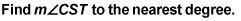2. A school is building a ramp for wheelchair access into the school. The ramp needs to be built from a point 20 feet away from the building and up to a door that is 10 feet off the ground. What is the degree measure that the ramp will make with the ground to the nearest degree?

1. 65 degrees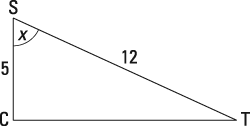In a right triangle, the following trigonometric proportions are true: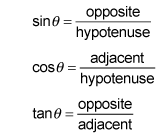In this triangle, you’re looking for information about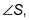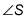and the hypotenuse. Therefore, use the cosine function: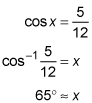2. 27 degrees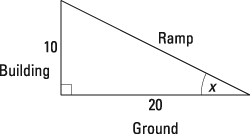In a right triangle, the following trigonometric proportions are true:In this triangle, you’re looking for information about the angle the ramp makes with the ground, and you’re given information about the side opposite it and the side adjacent to it. Therefore, use the tangent function: